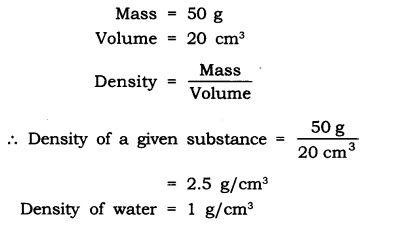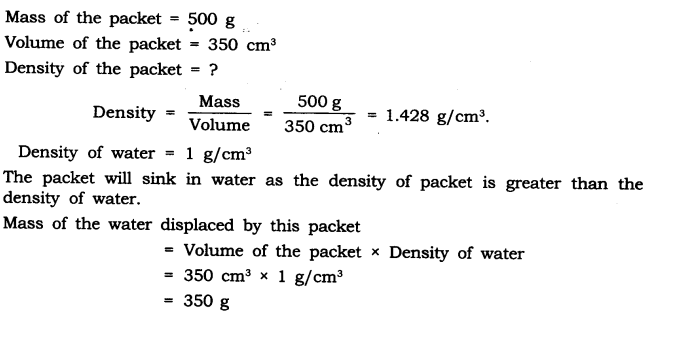# NCERT Solutions for Class 9 Science Chapter 10 Gravitation

NCERT Solutions for Class 9 Science Chapter 10 Gravitation

Class 9 Science NCERT Textbook Page 134

Question:- 1. State the universal law of gravitation.

Answer:-  According to the universal law of gravity, every object in the universe attracts every other object through a force known as gravitational attraction. The force acting between two objects is proportional to the product of their masses and inversely proportional to the square of their distance.

Question:- 2. Write the formula to find the magnitude of the gravitational force between the earth and an object on the surface of the earth.

Answer:- F = G Mm/R2

Where, F=  force of attraction between the earth and an object on the surface of the earth

m = Mass of the object on the surface of the earth.

M = Mass of the earth.

R = Distance between the earth’s centre and object.

Class 9 Science NCERT Textbook Page 136

Question:- 1. What do you mean by free fall?

Answer:- Every thing is attracted to the earth’s centre by gravity. When an object is dropped from a specific height, gravitational force causes it to fall towards the Earth’s surface. The motion of an object in this manner is known as free fall.

Question:- 2. What do you mean by acceleration due to gravity?

Answer:- The velocity of an object changes when it falls freely towards the earth’s surface from a specific height. This change in velocity causes the item to accelerate, which is known as acceleration due to gravity, and is symbolised by the letter ‘g’.

g=9.8 m/s2 is the value of gravity acceleration.

Class 9 Science NCERT Textbook Page 138

Question:- 1. What are the differences between the mass of an object and its weight?

 Mass Weight Mass is the quantity of matter contained in the body. Weight is the force of gravity acting on the body. It is the measure of inertia of the body. It is the measure of gravity. It only has magnitude. It has magnitude as well as direction. Mass is a constant quantity. Weight is not a constant quantity. It is different at different places. Its SI unit is kilogram (kg). Its SI unit is the same as the SI unit of force, i.e., Newton (N).

Question:- 2. Why is the weight of an object on the moon 1/6th its weight on the earth?

Answer:- The moon’s mass is 1/100 that of the earth, while its radius is 1/4 that of the earth. As a result, the moon’s gravitational attraction is about one-sixth that of the Earth. As a result, the weight of an object on the moon is 1/6 that of an object on Earth.

Class 9 Science NCERT Textbook Page 141

Question:- 1. Why is it difficult to hold a school bag having a strap made of a thin and strong string?

Answer:-  Because the force exerted by a thin and strong string is distributed over a smaller area, the force applied by the bag is more, the pressure imposed on the body by thin straps is greater, and thus the pain is greater.

As pressure is inversely related to area, pressure rises when the area is reduced.

Question:- 2. What do you mean by buoyancy?

Answer:-   Upthrust or buoyancy refers to the upward force exerted by any fluid (liquid, gas) on an object.

Question:- 3. Why does an object float or sink when placed on the surface of water?

Answer:-  When an object is placed on the surface of water, its density determines whether it will float or sink. When the density of an object is less than that of water, the object will floats and if its density is greater than the density of water then it will sink.

Class 9 Science NCERT Textbook Page 142

Question:- 1. You find your mass to be 42 kg on a weighing machine. Is your mass more or less than 42 kg?

Answer:-  The weighing machine actually measures the body’s weight as the acceleration due to gravity, or ‘g,’ acts on it. As a result, a weighing machine’s mass reading of 42 kg corresponds to the body’s real mass. It remains the same since mass is the quantity of inertia.

Question:- 2. You have a bag of cotton and an iron bar, each indicating a mass of 100 kg when measured on a weighing machine. In reality, one is heavier than other. Can you say which one is heavier and why?

Answer:- The heaviness of the bag can be given by density

Density= mass/volume

Cotton and iron bags have the same mass. However, the cotton bag has a larger volume than the iron bag. As a result, density and volume are inversely proportionate. The iron bag will be larger.

# Exercises

Question:- 1. How does the force of gravitation between two objects change when the distance between them is reduced to half ?

Answer:- Because the gravitational force between two items is inversely proportional to their square distance, if the distance between them is cut in half, the gravitational force will increase by four times.

Question:- 2. Gravitational force acts on all objects in proportion to their masses. Why then, a heavy object does not fall faster than a light object?

Answer:- All objects fall on earth with constant acceleration which is called acceleration due to gravity (in the absence of air resistances). It is constant and does not depend upon the mass of an object. As a result, heavy objects do not fall faster than light objects.

Question:- 3. What is the magnitude of the gravitational force between the earth and a 1 kg object on its surface? (Mass of the earth is 6 × 1024 kg and radius of the earth is 6.4 × 106 m.)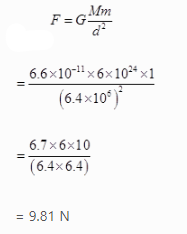Question:- 4. The earth and the moon are attracted to each other by gravitational force. Does the earth attract the moon with a force that is greater or smaller or the same as the force with which the moon attracts the earth? Why?

Answer:- Two objects attract each other with equal power but in opposite directions, according to the universal law of gravitation. The moon is attracted to the Earth with the same power as the moon is attracted to the Earth.

Question:- 5. If the moon attracts the earth, why does the earth not move towards the moon?

Answer:- The gravitational forces between the Earth and the moon are equivalent. The Earth, on the other hand, has a significantly larger mass than the moon. As a result, it accelerates at a slower pace than the moon as it approaches the Earth. As a result, the Earth does not move closer to the moon.

Question:- 6. What happens to the force between two objects, if

(i) the mass of one object is doubled?

(ii) the distance between the objects is doubled and tripled?

(iii) the masses of both objects are doubled?

Answer:- (i) the force between two objects will be doubled.

(ii) the force between two objects will become 1/4th and 1/9th of the present force.

(iii) the force between two objects will become four times the present force.

Question:- 7. What is the importance of universal law of gravitation?

Answer:- Many previously unconnected events are explained by the universal law of gravitation:

(i) The moon’s rotation around the earth

(ii) Gravity’s influence on the weight of the body, which holds us on the ground

(iii) The moon, and thus the Sun, cause the tides.

(iv) Planetary rotation around the Sun

Question:- 8. What is the acceleration of free fall?

Answer:- The acceleration gained by an object due to gravitational force is known as acceleration due to gravity. All bodies on Earth are subjected to a downward pull of gravity caused by the Earth’s mass. The acceleration of freely falling objects is used to calculate the Earth’s gravity. The acceleration of gravity at the Earth’s surface is 9.8 ms-2, which is symbolised by the letter ‘g.’ As a result, an object’s speed rises by around 9.8 metres per second for every second it is in free fall.

Question:- 9. What do we call the gravitational force between the earth and an object?

Answer:- Weight is the gravitational force between the earth and an object. The product of the acceleration due to gravity and the mass of the object is the weight.

Question:- 10. Amit buys few grams of gold at the poles as per the instruction of one of his friends. He hands over the same when he meets him at the equator. Will the friend agree with the weight of gold bought? If not, why? [Hint: The value of g is greater at the poles than at the equator.]

Answer:- At the poles, the value of gis is higher than at the equator. As a result, gold at the equator is lighter than gold at the poles. As a result, Amit’s friend will disagree with the gold’s weight.

Question:- 11. Why will a sheet of paper fall slower than one that is crumpled into a ball?

Answer:- The density of a sheet of paper increases when it is shattered into a ball. As a result, it has less resistance to falling through the air and falls faster than a sheet of paper.

Question:- 12. Gravitational force on the surface of the moon is only 1/6 as strong as gravitational force on the earth. What is the weight in newtons of a 10 kg object on the moon and on the earth?

Acceleration due to earth’s gravity = ge or g = 9.8 m/s2

Object’s mass, m = 10 kg

Acceleration due to moon gravity = gm

Weight on the earth= We

Weight on the moon = Wm

Weight = mass x gravity

gm = (1/6) ge (given)

So Wm = m gm = m x (1/6) ge

Wm = 10 x (1/6) x 9.8 = 16.34 N

We = m x ge = 10 x 9.8

We = 98N

Question:- 13. A ball is thrown vertically upwards with a velocity of 49 m/s.Calculate

(i) the maximum height to which it rises,

(ii) the total time it takes to return to the surface of the earth.

Initial velocity u = 49 m/s

Final speed v at maximum height = 0

Acceleration due to earth gravity g = -9.8 m/s2 (thus negative as ball is thrown up).

By third equation of motion,

2gH = v2 – u2

2 × (- 9.8) × H = 0 – (49)2

– 19.6 H = – 2401

H = 122.5 m

Total time T = Time to ascend (Ta) + Time to descend (Td)

v = u + gt

0 = 49 + (-9.8) x Ta

Ta = (49/9.8) = 5 s

Also, Td = 5 s

Therefore T = Ta + Td

T = 5 + 5

T = 10 s

Question:- 14. A stone is released from the top of a tower of height 19.6 m. Calculate its final velocity just before touching the ground.

Initial velocity

u = 0

Tower height = total distance = 19.6m

g = 9.8 m/s2

Consider third equation of motion

v2 = u2 + 2gs

v2 = 0 + 2 × 9.8 × 19.6

v2 = 384.16

v = √(384.16)

v = 19.6m/s

Question:-15. A stone is thrown vertically upward with an initial velocity of 40 m/s. Taking g = 10 m/s2, find the maximum height reached by the stone. What is the net displacement and the total distance covered by the stone?

Initial velocity u = 40m/s

g = 10 m/s2

Max height final velocity = 0

Consider third equation of motion

v2 = u2 – 2gs [negative as the object goes up]

0 = (40)2 – 2 x 10 x s

s = (40 x 40) / 20

Maximum height s = 80m

Total Distance = s + s = 80 + 80

Total Distance = 160m

Total displacement = 0 (The first point is the same as the last point)

Question:- 16. Calculate the force of gravitation between the earth and the Sun, given that the mass of the earth = 6 × 1024 kg and of the Sun = 2 × 1030 kg. The average distance between the two is 1.5 × 1011 m.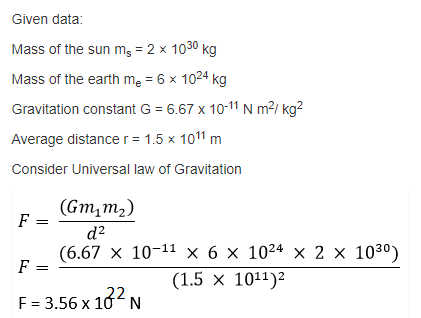Question:- 17. A stone is allowed to fall from the top of a tower 100 m high and at the same time another stone is projected vertically upwards from the ground with a velocity of 25 m/s. Calculate when and where the two stones will meet.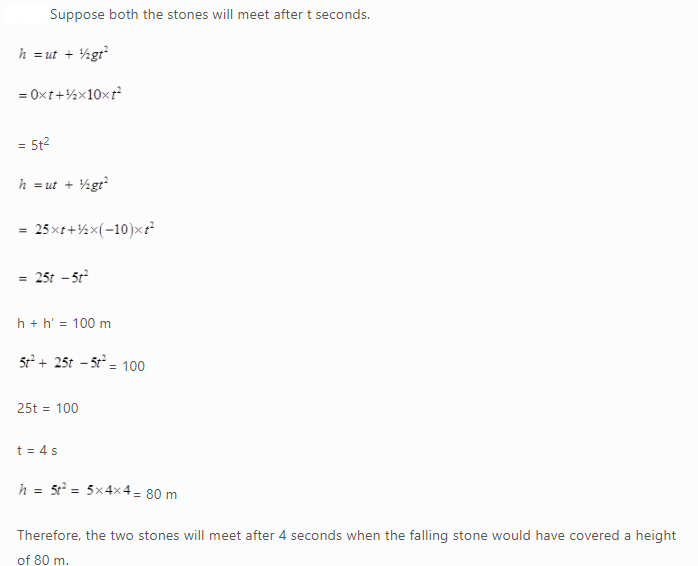Question:- 18. A ball thrown up vertically returns to the thrower after 6 s. Find

(a) the velocity with which it was thrown up,

(b) the maximum height it reaches, and

(c) its position after 4 s.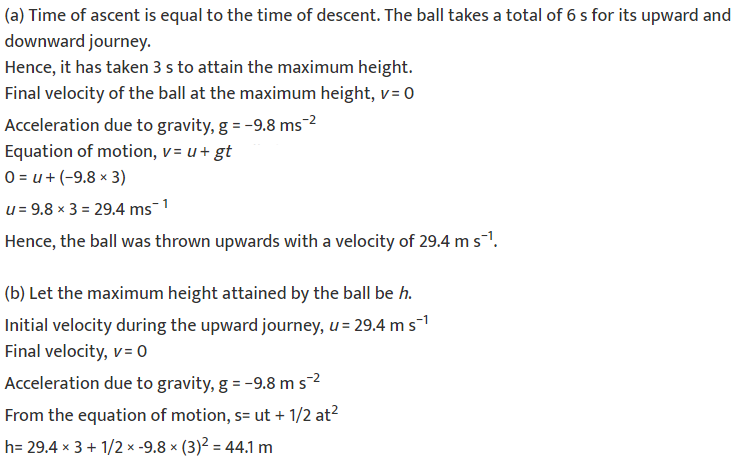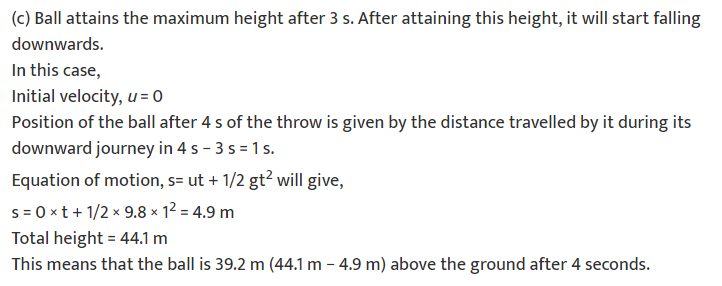Question:- 19. In what direction does the buoyant force on an object immersed in a liquid act?

Answer:- The buoyant force on an object immersed in a liquid acts opposite to the direction of the force exerted by the object, i.e. in upward direction.

Question:- 20. Why does a block of plastic released under water come up to the surface of water?

Answer:- Plastic has a lower density than water and the buoyant force exerted by water on the plastic block is greater than the force exerted by plastic on the water. Hence, when a block of plastic released under water come up to the surface of water.

Question:- 21. The volume of 50 g of a substance is 20 cm3. If the density of water is 1 g cm–3, will the substance float or sink?